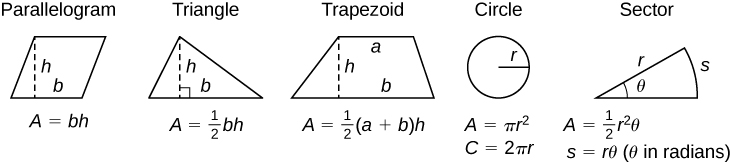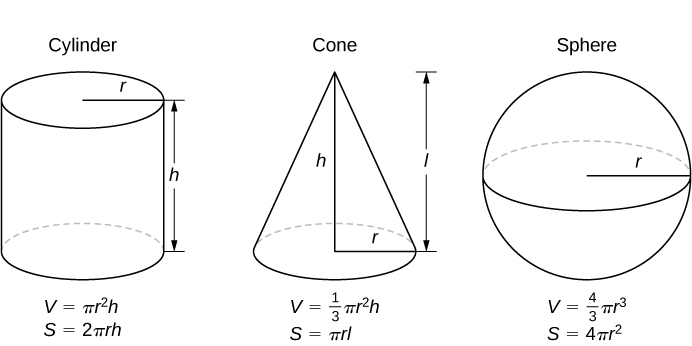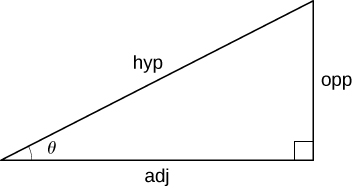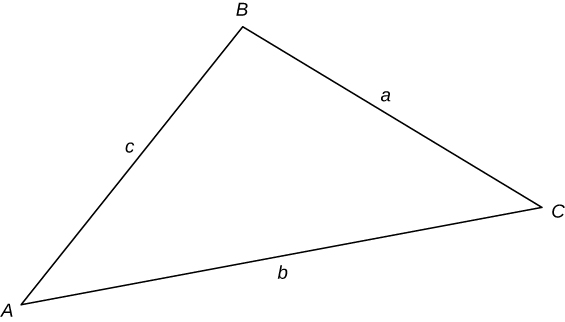# 0.3 Review of pre-calculus

 Page 1 / 1

## Formulas from geometry

$A=\text{area},$ $V=\text{Volume},\phantom{\rule{0.2em}{0ex}}\text{and}$ $S=\text{lateral surface area}$## Laws of exponents

$\begin{array}{ccccccccccccc}\hfill {x}^{m}{x}^{n}& =\hfill & {x}^{m+n}\hfill & & & \hfill \frac{{x}^{m}}{{x}^{n}}& =\hfill & {x}^{m-n}\hfill & & & \hfill {\left({x}^{m}\right)}^{n}& =\hfill & {x}^{mn}\hfill \\ \hfill {x}^{\text{−}n}& =\hfill & \frac{1}{{x}^{n}}\hfill & & & \hfill {\left(xy\right)}^{n}& =\hfill & {x}^{n}{y}^{n}\hfill & & & \hfill {\left(\frac{x}{y}\right)}^{n}& =\hfill & \frac{{x}^{n}}{{y}^{n}}\hfill \\ \hfill {x}^{1\text{/}n}& =\hfill & \sqrt[n]{x}\hfill & & & \hfill \sqrt[n]{xy}& =\hfill & \sqrt[n]{x}\sqrt[n]{y}\hfill & & & \hfill \sqrt[n]{\frac{x}{y}}& =\hfill & \frac{\sqrt[n]{x}}{\sqrt[n]{y}}\hfill \\ \hfill {x}^{m\text{/}n}& =\hfill & \sqrt[n]{{x}^{m}}={\left(\sqrt[n]{x}\right)}^{m}\hfill & & & & & & & & & & \end{array}$

## Special factorizations

$\begin{array}{ccc}\hfill {x}^{2}-{y}^{2}& =\hfill & \left(x+y\right)\left(x-y\right)\hfill \\ \hfill {x}^{3}+{y}^{3}& =\hfill & \left(x+y\right)\left({x}^{2}-xy+{y}^{2}\right)\hfill \\ \hfill {x}^{3}-{y}^{3}& =\hfill & \left(x-y\right)\left({x}^{2}+xy+{y}^{2}\right)\hfill \end{array}$

If $a{x}^{2}+bx+c=0,$ then $x=\frac{\text{−}b±\sqrt{{b}^{2}-4ca}}{2a}.$

## Binomial theorem

${\left(a+b\right)}^{n}={a}^{n}+\left(\begin{array}{l}n\\ 1\end{array}\right){a}^{n-1}b+\left(\begin{array}{l}n\\ 2\end{array}\right){a}^{n-2}{b}^{2}+\cdots +\left(\begin{array}{c}n\\ n-1\end{array}\right)a{b}^{n-1}+{b}^{n},$

where $\left(\begin{array}{l}n\\ k\end{array}\right)=\frac{n\left(n-1\right)\left(n-2\right)\cdots \left(n-k+1\right)}{k\left(k-1\right)\left(k-2\right)\cdots 3\cdot 2\cdot 1}=\frac{n!}{k!\left(n-k\right)!}$

## Right-angle trigonometry

$\begin{array}{cccc}\text{sin}\phantom{\rule{0.1em}{0ex}}\theta =\frac{\text{opp}}{\text{hyp}}\hfill & & & \text{csc}\phantom{\rule{0.1em}{0ex}}\theta =\frac{\text{hyp}}{\text{opp}}\hfill \\ \text{cos}\phantom{\rule{0.1em}{0ex}}\theta =\frac{\text{adj}}{\text{hyp}}\hfill & & & \text{sec}\phantom{\rule{0.1em}{0ex}}\theta =\frac{\text{hyp}}{\text{adj}}\hfill \\ \text{tan}\phantom{\rule{0.1em}{0ex}}\theta =\frac{\text{opp}}{\text{adj}}\hfill & & & \text{cot}\phantom{\rule{0.1em}{0ex}}\theta =\frac{\text{adj}}{\text{opp}}\hfill \end{array}$## Trigonometric functions of important angles

 $\theta$ $\text{Radians}$ $\text{sin}\phantom{\rule{0.1em}{0ex}}\theta$ $\text{cos}\phantom{\rule{0.1em}{0ex}}\theta$ $\text{tan}\phantom{\rule{0.1em}{0ex}}\theta$ $0\text{°}$ $0$ $0$ $1$ $0$ $30\text{°}$ $\text{π}\text{/}\text{6}$ $1\text{/}2$ $\sqrt{3}\text{/}2$ $\sqrt{3}\text{/}3$ $45\text{°}$ $\text{π}\text{/}\text{4}$ $\sqrt{2}\text{/}2$ $\sqrt{2}\text{/}2$ $1$ $60\text{°}$ $\text{π}\text{/}\text{3}$ $\sqrt{3}\text{/}2$ $1\text{/}2$ $\sqrt{3}$ $90\text{°}$ $\text{π}\text{/}2$ $1$ $0$ —

## Fundamental identities

$\begin{array}{cccccccc}\hfill {\text{sin}}^{2}\theta +{\text{cos}}^{2}\theta & =\hfill & 1\hfill & & & \hfill \text{sin}\left(\text{−}\phantom{\rule{0.1em}{0ex}}\theta \right)& =\hfill & \text{−}\text{sin}\phantom{\rule{0.1em}{0ex}}\theta \hfill \\ \hfill 1+{\text{tan}}^{2}\theta & =\hfill & {\text{sec}}^{2}\theta \hfill & & & \hfill \text{cos}\left(\text{−}\phantom{\rule{0.1em}{0ex}}\theta \right)& =\hfill & \text{cos}\phantom{\rule{0.1em}{0ex}}\theta \hfill \\ \hfill 1+{\text{cot}}^{2}\theta & =\hfill & {\text{csc}}^{2}\theta \hfill & & & \hfill \text{tan}\left(\text{−}\phantom{\rule{0.1em}{0ex}}\theta \right)& =\hfill & \text{−}\text{tan}\phantom{\rule{0.1em}{0ex}}\theta \hfill \\ \hfill \text{sin}\left(\frac{\pi }{2}-\theta \right)& =\hfill & \text{cos}\phantom{\rule{0.1em}{0ex}}\theta \hfill & & & \hfill \text{sin}\left(\theta +2\pi \right)& =\hfill & \text{sin}\phantom{\rule{0.1em}{0ex}}\theta \hfill \\ \hfill \text{cos}\left(\frac{\pi }{2}-\theta \right)& =\hfill & \text{sin}\phantom{\rule{0.1em}{0ex}}\theta \hfill & & & \hfill \text{cos}\left(\theta +2\pi \right)& =\hfill & \text{cos}\phantom{\rule{0.1em}{0ex}}\theta \hfill \\ \hfill \text{tan}\left(\frac{\pi }{2}-\theta \right)& =\hfill & \text{cot}\phantom{\rule{0.1em}{0ex}}\theta \hfill & & & \hfill \text{tan}\left(\theta +\pi \right)& =\hfill & \text{tan}\phantom{\rule{0.1em}{0ex}}\theta \hfill \end{array}$

## Law of sines

$\frac{\text{sin}\phantom{\rule{0.1em}{0ex}}A}{a}=\frac{\text{sin}\phantom{\rule{0.1em}{0ex}}B}{b}=\frac{\text{sin}\phantom{\rule{0.1em}{0ex}}C}{c}$## Law of cosines

$\begin{array}{ccc}\hfill {a}^{2}& =\hfill & {b}^{2}+{c}^{2}-2bc\phantom{\rule{0.2em}{0ex}}\text{cos}\phantom{\rule{0.2em}{0ex}}A\hfill \\ \hfill {b}^{2}& =\hfill & {a}^{2}+{c}^{2}-2ac\phantom{\rule{0.2em}{0ex}}\text{cos}\phantom{\rule{0.2em}{0ex}}B\hfill \\ \hfill {c}^{2}& =\hfill & {a}^{2}+{b}^{2}-2ab\phantom{\rule{0.2em}{0ex}}\text{cos}\phantom{\rule{0.2em}{0ex}}C\hfill \end{array}$

$\begin{array}{ccc}\hfill \text{sin}\phantom{\rule{0.2em}{0ex}}\left(x+y\right)& =\hfill & \text{sin}\phantom{\rule{0.2em}{0ex}}x\phantom{\rule{0.2em}{0ex}}\text{cos}\phantom{\rule{0.2em}{0ex}}y+\text{cos}\phantom{\rule{0.2em}{0ex}}x\phantom{\rule{0.2em}{0ex}}\text{sin}\phantom{\rule{0.2em}{0ex}}y\hfill \\ \hfill \text{sin}\phantom{\rule{0.1em}{0ex}}\left(x-y\right)& =\hfill & \text{sin}\phantom{\rule{0.2em}{0ex}}x\phantom{\rule{0.2em}{0ex}}\text{cos}\phantom{\rule{0.2em}{0ex}}y-\text{cos}\phantom{\rule{0.2em}{0ex}}x\phantom{\rule{0.2em}{0ex}}\text{sin}\phantom{\rule{0.2em}{0ex}}y\hfill \\ \hfill \text{cos}\phantom{\rule{0.1em}{0ex}}\left(x+y\right)& =\hfill & \text{cos}\phantom{\rule{0.2em}{0ex}}x\phantom{\rule{0.2em}{0ex}}\text{cos}\phantom{\rule{0.2em}{0ex}}y-\text{sin}\phantom{\rule{0.2em}{0ex}}x\phantom{\rule{0.2em}{0ex}}\text{sin}\phantom{\rule{0.2em}{0ex}}y\hfill \\ \hfill \text{cos}\phantom{\rule{0.1em}{0ex}}\left(x-y\right)& =\hfill & \text{cos}\phantom{\rule{0.2em}{0ex}}x\phantom{\rule{0.2em}{0ex}}\text{cos}\phantom{\rule{0.2em}{0ex}}y+\text{sin}\phantom{\rule{0.2em}{0ex}}x\phantom{\rule{0.2em}{0ex}}\text{sin}\phantom{\rule{0.2em}{0ex}}y\hfill \\ \hfill \text{tan}\phantom{\rule{0.1em}{0ex}}\left(x+y\right)& =\hfill & \frac{\text{tan}\phantom{\rule{0.2em}{0ex}}x+\text{tan}y}{1-\text{tan}\phantom{\rule{0.2em}{0ex}}x\phantom{\rule{0.2em}{0ex}}\text{tan}y}\hfill \\ \hfill \text{tan}\left(x-y\right)& =\hfill & \frac{\text{tan}\phantom{\rule{0.2em}{0ex}}x-\text{tan}y}{1+\text{tan}\phantom{\rule{0.2em}{0ex}}x\phantom{\rule{0.2em}{0ex}}\text{tan}y}\hfill \end{array}$

## Double-angle formulas

$\begin{array}{ccc}\hfill \text{sin}\phantom{\rule{0.2em}{0ex}}2x& =\hfill & 2\phantom{\rule{0.1em}{0ex}}\text{sin}\phantom{\rule{0.2em}{0ex}}x\phantom{\rule{0.2em}{0ex}}\text{cos}\phantom{\rule{0.2em}{0ex}}x\hfill \\ \hfill \text{cos}\phantom{\rule{0.2em}{0ex}}2x& =\hfill & {\text{cos}}^{2}x-{\text{sin}}^{2}x=2\phantom{\rule{0.1em}{0ex}}{\text{cos}}^{2}x-1=1-2\phantom{\rule{0.1em}{0ex}}{\text{sin}}^{2}x\hfill \\ \hfill \text{tan}\phantom{\rule{0.2em}{0ex}}2x& =\hfill & \frac{2\phantom{\rule{0.1em}{0ex}}\text{tan}\phantom{\rule{0.2em}{0ex}}x}{1-{\text{tan}}^{2}x}\hfill \end{array}$

## Half-angle formulas

$\begin{array}{ccc}\hfill {\text{sin}}^{2}x& =\hfill & \frac{1-\text{cos}\phantom{\rule{0.2em}{0ex}}2x}{2}\hfill \\ \hfill {\text{cos}}^{2}x& =\hfill & \frac{1+\text{cos}\phantom{\rule{0.2em}{0ex}}2x}{2}\hfill \end{array}$

f(x) = x-2 g(x) = 3x + 5 fog(x)? f(x)/g(x)
fog(x)= f(g(x)) = x-2 = 3x+5-2 = 3x+3 f(x)/g(x)= x-2/3x+5
diron
pweding paturo nsa calculus?
jimmy
how to use fundamental theorem to solve exponential
find the bounded area of the parabola y^2=4x and y=16x
what is absolute value means?
Chicken nuggets
Hugh
🐔
MM
🐔🦃 nuggets
MM
(mathematics) For a complex number a+bi, the principal square root of the sum of the squares of its real and imaginary parts, √a2+b2 . Denoted by | |. The absolute value |x| of a real number x is √x2 , which is equal to x if x is non-negative, and −x if x is negative.
Ismael
find integration of loge x
find the volume of a solid about the y-axis, x=0, x=1, y=0, y=7+x^3
how does this work
Can calculus give the answers as same as other methods give in basic classes while solving the numericals?
log tan (x/4+x/2)
Rohan
Rohan
y=(x^2 + 3x).(eipix)
Claudia
Ismael
A Function F(X)=Sinx+cosx is odd or even?
neither
David
Neither
Lovuyiso
f(x)=1/1+x^2 |=[-3,1]
apa itu?
fauzi
determine the area of the region enclosed by x²+y=1,2x-y+4=0
Hi
MP
Hi too
Vic
hello please anyone with calculus PDF should share
Which kind of pdf do you want bro?
Aftab
hi
Abdul
can I get calculus in pdf
Abdul
explain for me
Usman
How to use it to slove fraction
Hello please can someone tell me the meaning of this group all about, yes I know is calculus group but yet nothing is showing up
Shodipo
You have downloaded the aplication Calculus Volume 1, tackling about lessons for (mostly) college freshmen, Calculus 1: Differential, and this group I think aims to let concerns and questions from students who want to clarify something about the subject. Well, this is what I guess so.
Jean
Im not in college but this will still help
nothing
how en where can u apply it
Migos
how can we scatch a parabola graph
Ok
Endalkachew
how can I solve differentiation?
with the help of different formulas and Rules. we use formulas according to given condition or according to questions
CALCULUS
For example any questions...
CALCULUS
v=(x,y) وu=(x,y ) ∂u/∂x* ∂x/∂u +∂v/∂x*∂x/∂v=1
log tan (x/4+x/2)
Rohan
what is the procedures in solving number 1?By Yasser IbrahimBy John GabrieliBy Lakeima RobertsBy OpenStaxBy Carly AllenBy OpenStaxBy OpenStaxBy JavaChamp TeamBy Szilárd JankóBy OpenStax